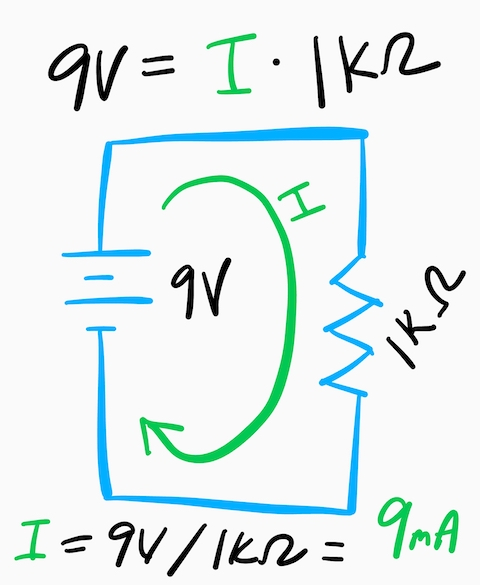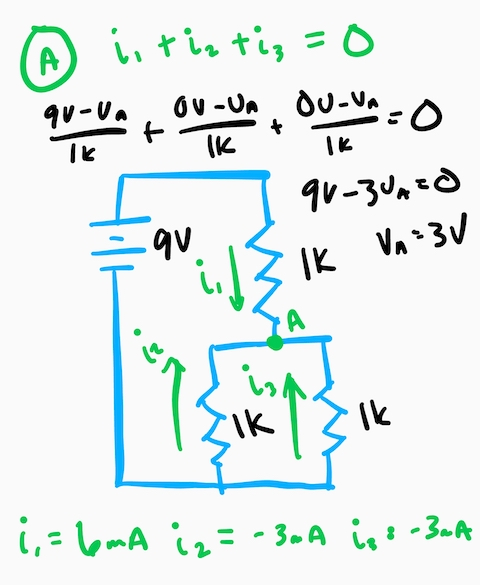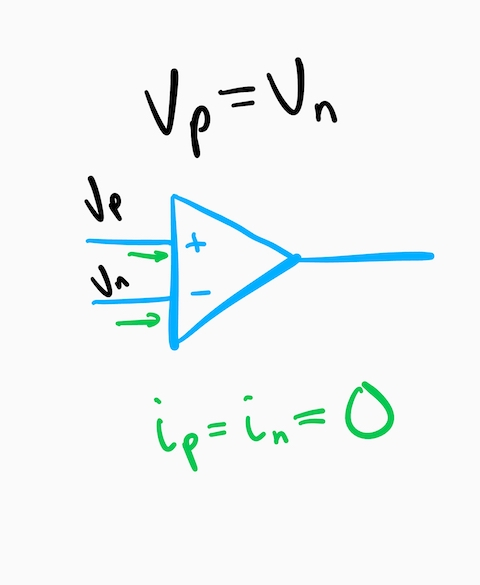Analyzing Op AmpsOperational amplifiers, or op-amps as they are affectionately known, are very useful when designing embedded systems and relatively easy-to-use. If you know just a few of the basics, you can design and analyze op-amps like a boss.

Ohm’s Law

The first principle to understand is ohm’s law which states that the voltage dropped across a resistor is equal to the resistance times the current (V=IR). Consider the circuit above.

A one kilo-ohm resistor is connected to a 9V battery. Using simple algebra, Ohm’s law can be used to solve for the current through the resistor which is 9mA.

Kirchhoff’s Current Law

The next important principle is Kirchhoff’s current law which states that the sum of alls currents into a node is zero. Consider the following circuit.

From Kirchhoff, the sum of currents into node A is zero. Please note, the positive current is defined as flowing in the direction of the arrow. If a particular current is negative, a positive current flows in the direction opposite the arrow.At node A, currents i1, i2, and i3 converge. Using Ohm’s law, we can substitute these values with equivalent V/R values. So, i1 becomes (9V-VA)/1k. Because the arrow is drawn from ground to node A, i2 becomes (0V-VA)/1k. Finally, i3 is substituted for (0V-VA)/1k. This equation leaves a single unknown value VA, which when solved results in VA = 3V.

Once VA is known values for i1, i2, and i3 are calculated to show 6mA + -3mA + -3mA = 0 just like Kirchhoff said it would.

Ideal Op-Amp Characteristics

The last principle to grasp for op-amp analysis is the ideal characteristics of an op-amp.

1. The voltage at the positive terminal is equal to the voltage at the negative terminal
2. No current flows in or out of the positive or negative terminal

These characteristics are illustrated in the image below.In reality, the voltage between the terminals is slightly different, and a tiny amount of current flows through the terminals. Because these values don’t have practical effects on the circuit, we can ignore them when analyzing op-amps.

Inverting Amplifier

When analyzing op-amps, we are tying to determine the relationship between the output voltage (VO) and the input voltage (VI). This relationship is typically expressed as a ratio of VO/VI known as the gain.

To analyze the gain of the amplifier shown, we apply Kirchhoff’s current law at the negative terminal. We then substitute voltage and resistance for current using Ohm’s law. Finally, we substitute values based on ideal op-amp characteristics and then calculate the gain using a little algebra.Wrap Up

That example was an easy one, but you can apply this method to many op-amp configurations.

X